# EuroEconomica, Vol 35, No 2 (2016)

A Mathematical Model of Working Time and Leisure

Cătălin Angelo Ioan1,, Gina Ioan2

Abstract: The paper determines the optimal balance in terms of the producer between working time and leisure. The structure equations of the model were formulated being resolved in a particular case.

Keywords: work time; leisure; productivity

JEL Classification: C02

1. The Supply and Demand of Individual Work

Each individual providing a paid employment has a limited time which can either assign to work (paid work) or leisure.

Although the desire to have as much leisure exists for most of people, they must devote many hours for work, for which they are paid in order to meet needs, which obviously include also the spending of leisure.

Any individual working income is determined by the number of hours worked and wage levels.

When income increases, meaning that each hour allocated to labor becomes more expensive, the individual can buy more goods and services. In this situation any hour of the 24 hours allocated to leisure means an hour less of the assigned work and fewer goods and services purchased. Rather, reducing leisure time increases the number of hours worked. How anyone can not work 24 hours out of 24 (otherwise he could not take advantage of the benefits that work provides), the opportunity cost of leisure is the goods and services sacrificed.

Considering individual who is willing to offer its workforce like a consumer, he has to choose between two normal goods (leisure and number of hours worked or wages and hours worked) different combinations but giving the same utility. It can be drawn the indifference curves for an individual to working hours and hourly wage received.

Theoretically, every rational being, and here we refer to the consumer, establish in every moment of its existence one or more programs of consumer and rational order his preferences. This is the point of view of the individual as the consumer.

On the other hand, in the production process, the individual is subjected to pressures exerted by the employer in order to obtain a bigger production (in fact, to obtain a maximal profit) and to grow his labor productivity growth.

Even if, in principle, the employer may be tempted to force the worker spending many hours to make an increasingly larger production, in reality, an increase of daily working time leads to a decrease in productivity.

Also, during leisure, the employee may complete knowledge by various methods. He can read professional literature, can surf the Internet (picking useful information), can watch documentaries at TV, activities that will lead to a greater or lesser increase its performance.

The question is therefore that of dosing by the employer of working time so as to be able to increase business performance from complementary activities carried out by the employee in his leisure.

2. The Equations of the Model

In what follows we shall use the following notations:

• T = total time = 24 h = constant;

• TW = work time (assuming that it is dependent on the production);

• TL = leisure;

• Q = daily production;

• W = wage;

• K = capital;

• L = number of workers;

• ATC = average total cost of production;

• c = capital price;

• = profit;

• p = selling price;

• wL = average labor productivity; wL=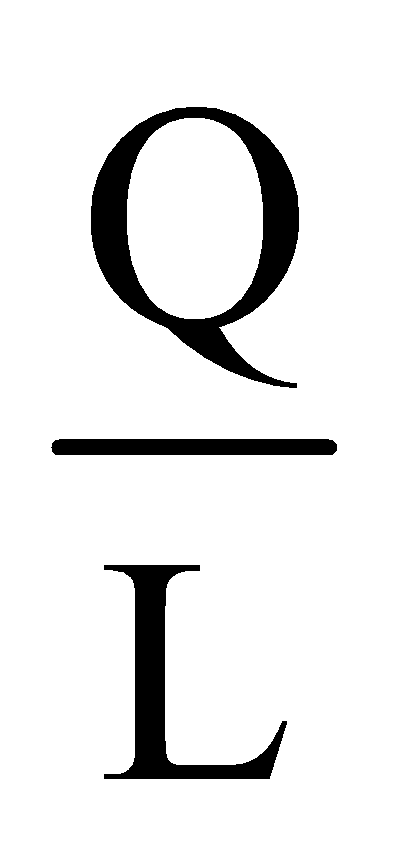;

• wK = average productivity of capital; wK=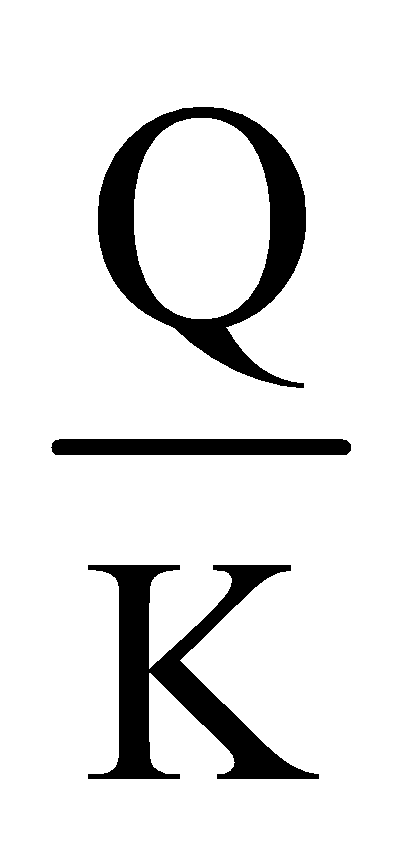.

The model's equations are:

(1) T=TW+TL;

From (1) follows: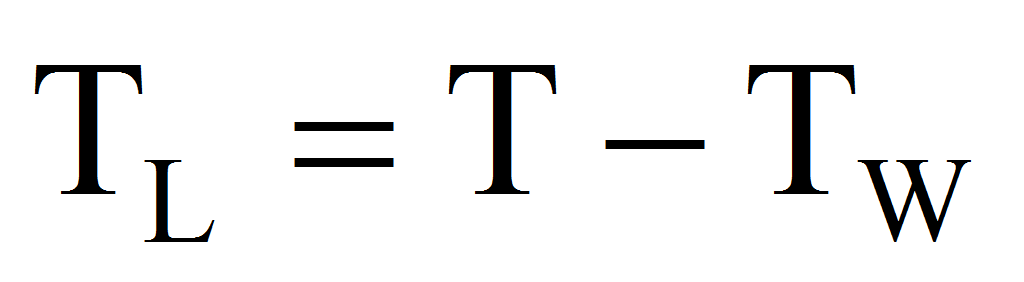from where:

(1’)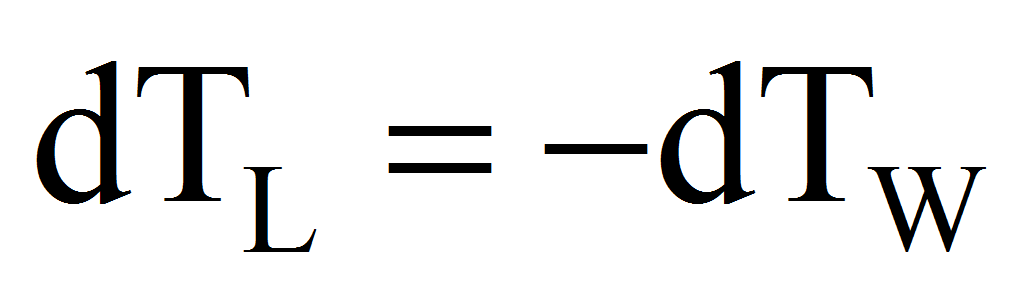(2)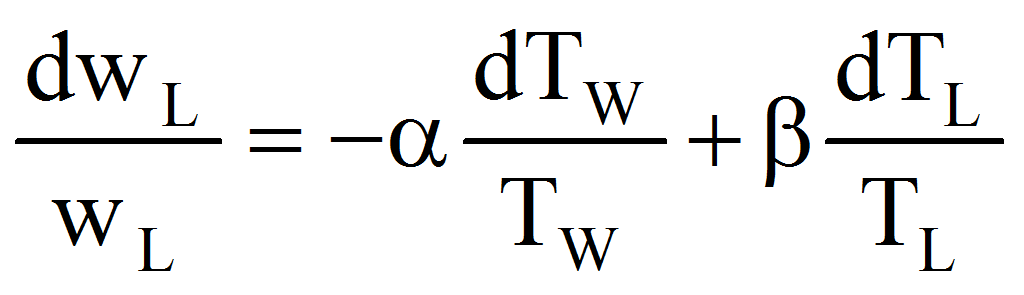where ,0, constants

Equation (2) expresses that the relative variation of average labor productivity depends decreasing of the relative variation of working time and increasing of the relative variation of leisure. The argument is that some of the free time is spent by workers either for training or for leisure or recreation which increases their performances.

Hence, integrating (as functions of Q):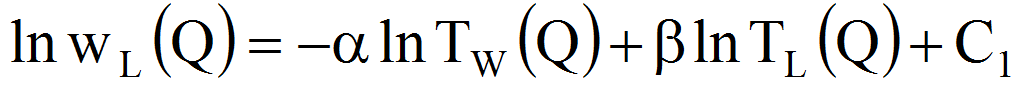therefore:

(2’)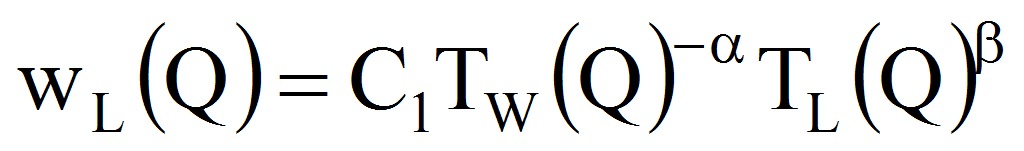where we note: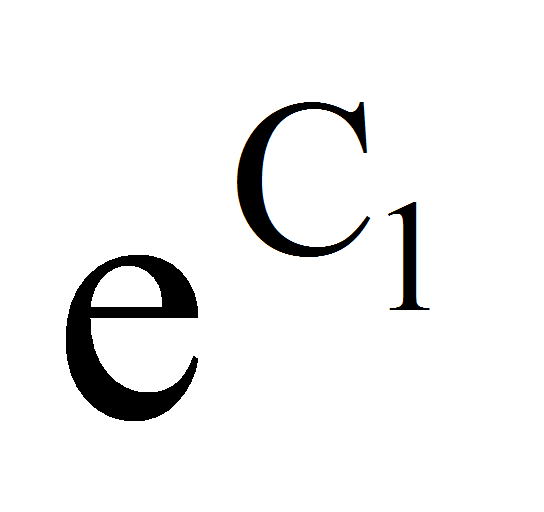with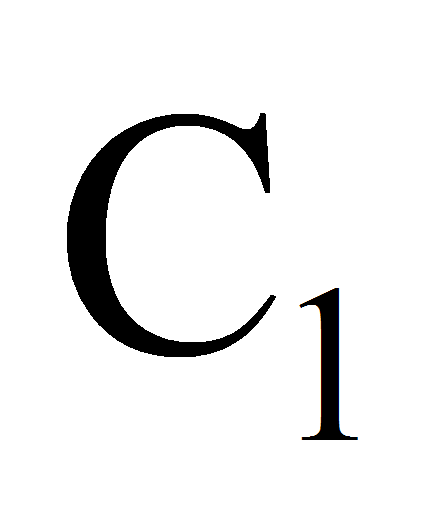0.

From (2’), (1) we have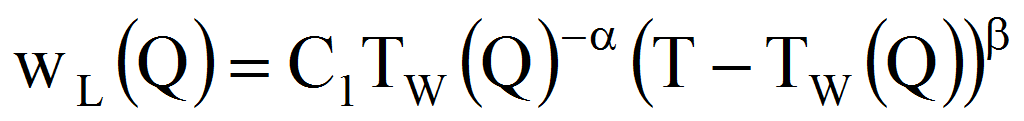.

Considering the fnction g:[0,T]R, g(x)=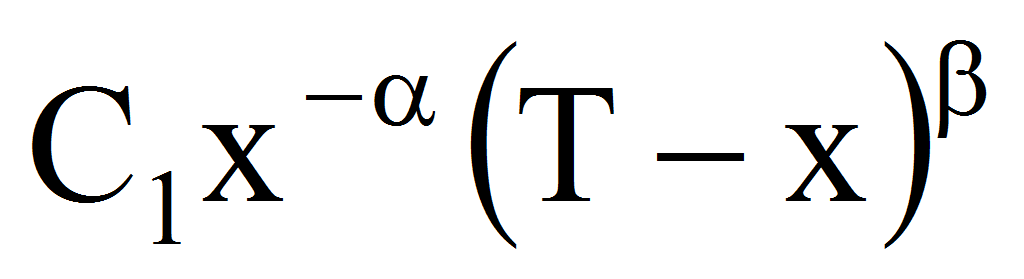we have: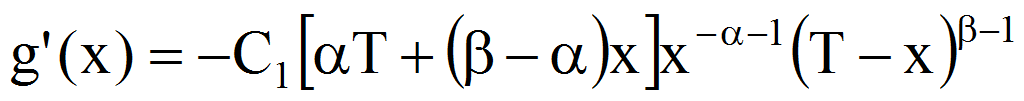x(0,T). If > we find that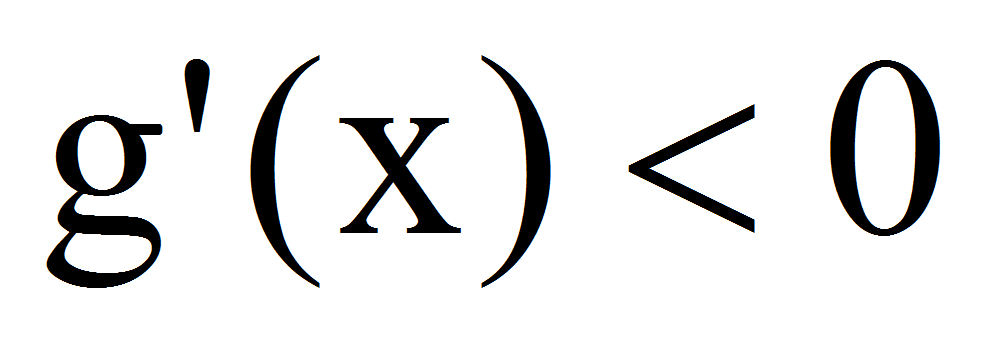therefore g is a decreasing function. As a consequence its maximum is attained at x=0 that is wL has a maximum for TW(Q)=0 which is absurd. Therefore we must have .

(3)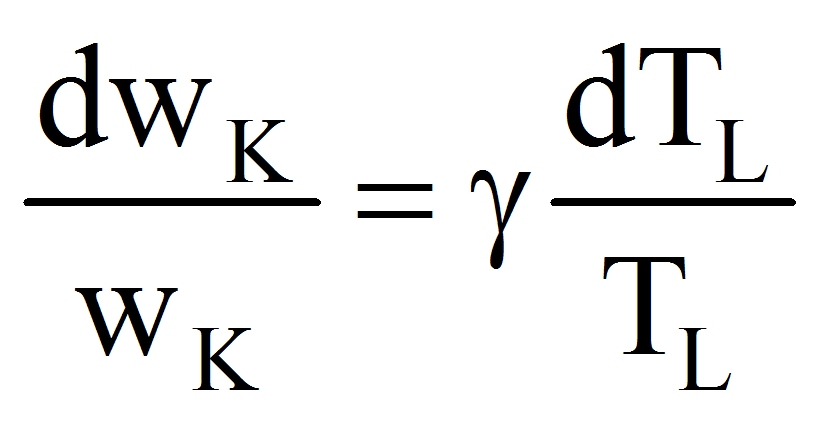where 0, constant

Equation (3) expresses that the relative variation of average productivity of capital depends on the relative variation of leisure (even with a small factor) because after personal training, workers can contribute to the growth of productivity capital through inventions or innovations.

Hence, integrating (as functions of Q):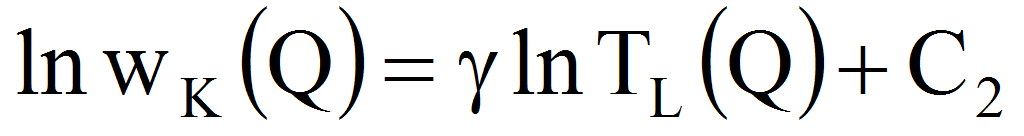therefore:

(3’)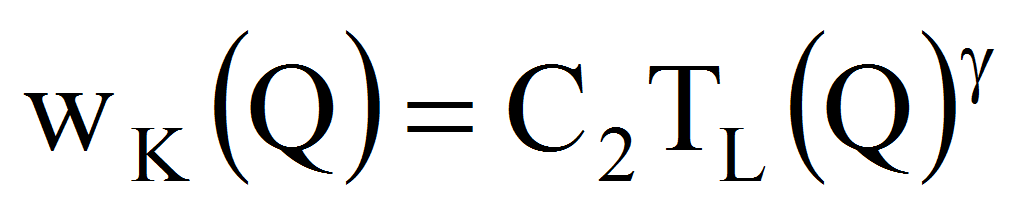where we note: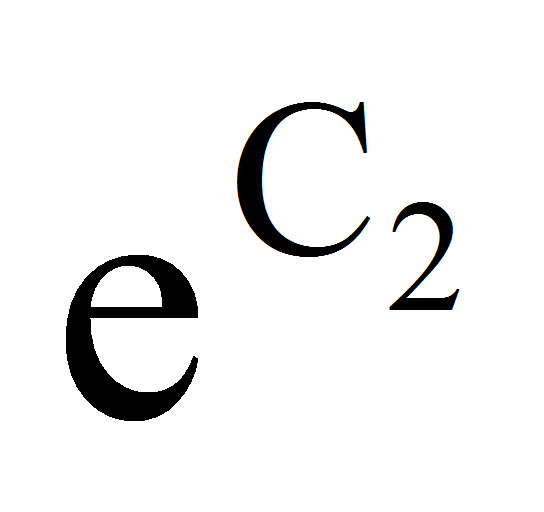with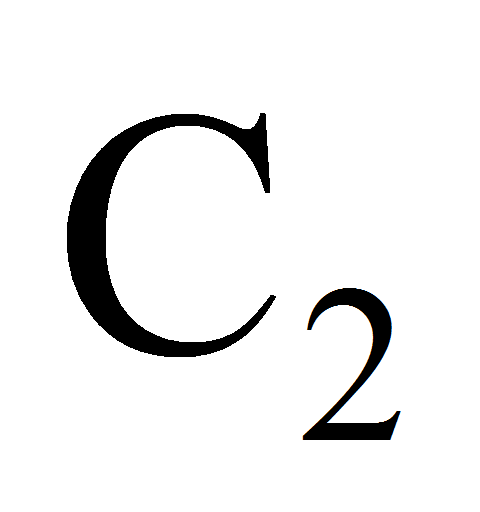0.

(4)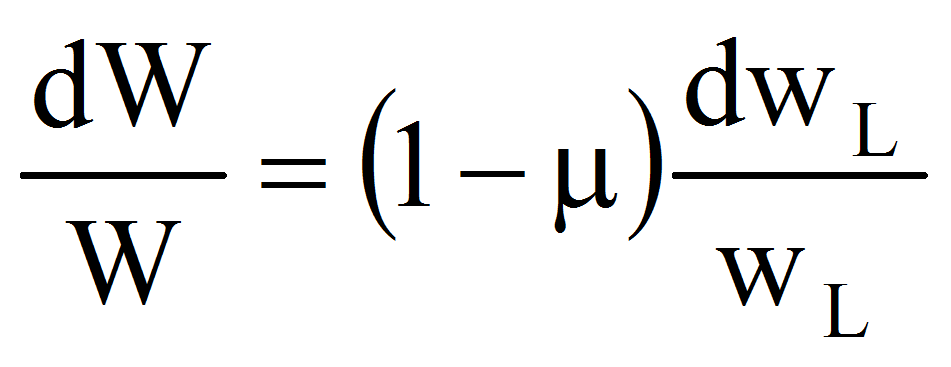, [0,1], constant

Equation (4) expresses that the relative variation of wage depends proportional on the relative variation of the average productivity of labor.

Hence, integrating (as functions of Q):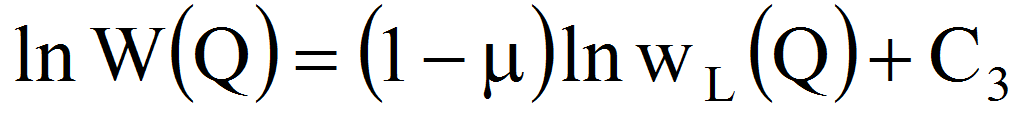therefore: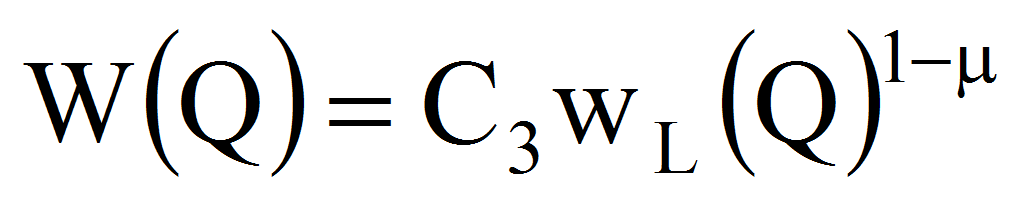where we note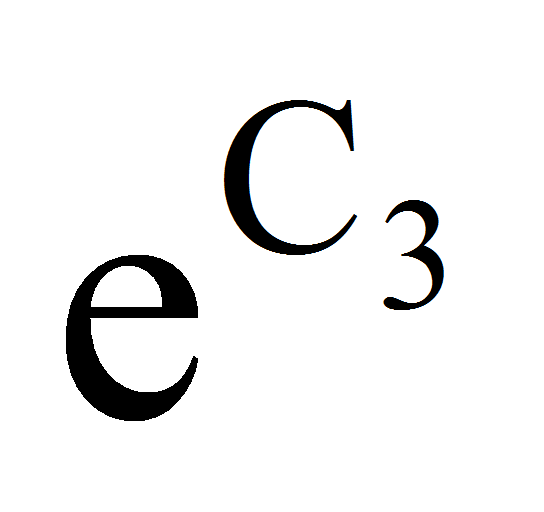with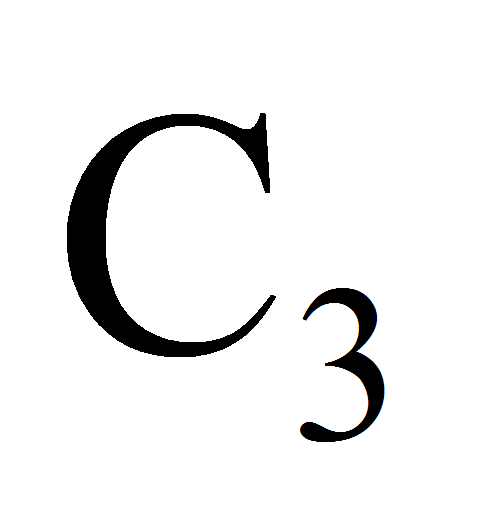0. From (2’) we have now:

(4’)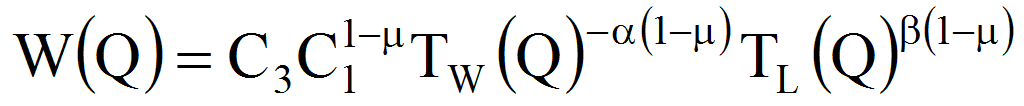(5) Q=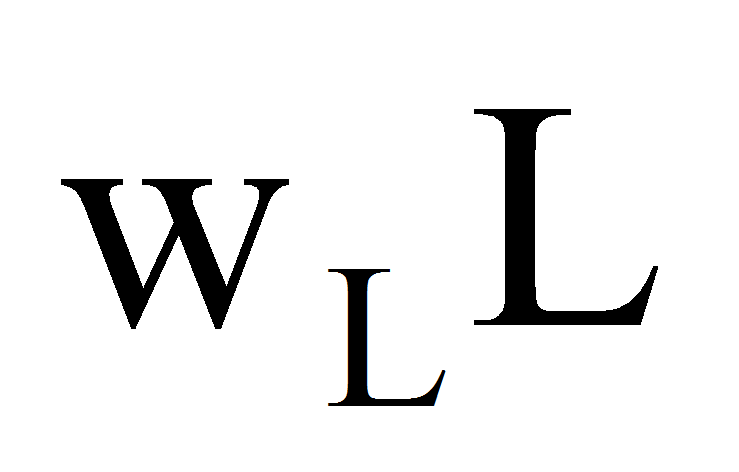=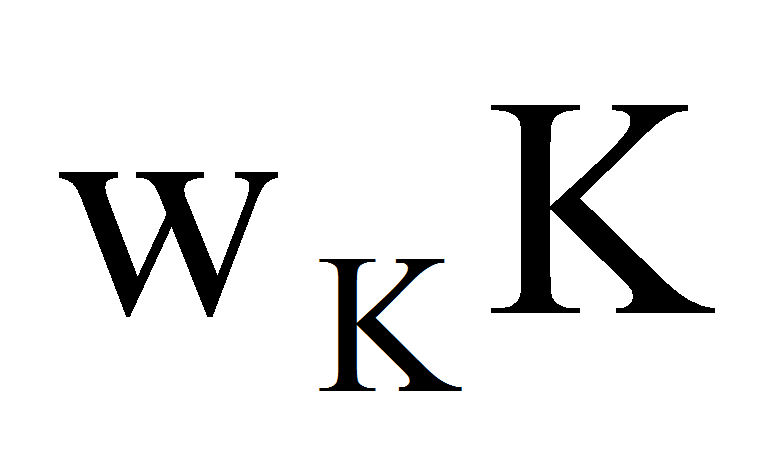(6)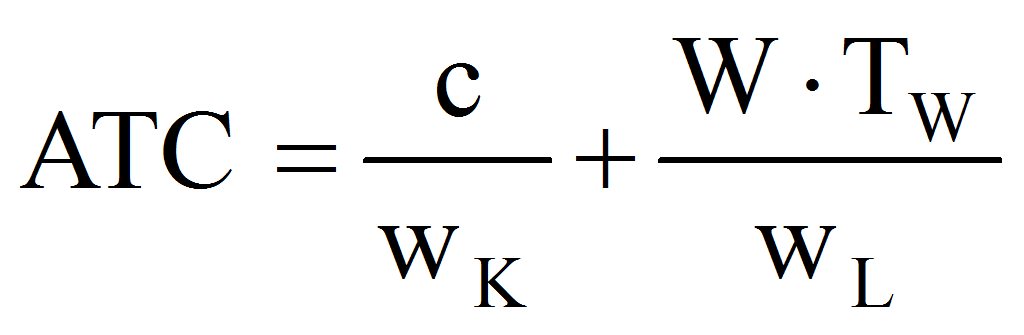The equation (6) comes from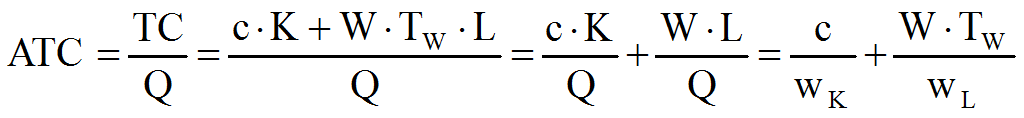.

From (6), (3’), (2’):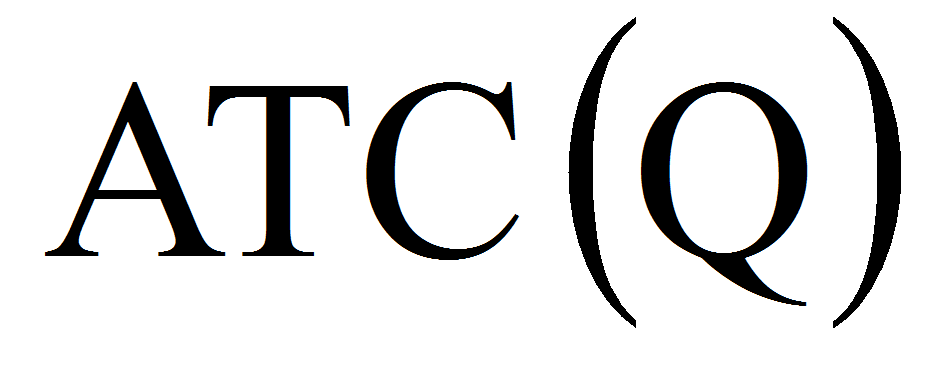=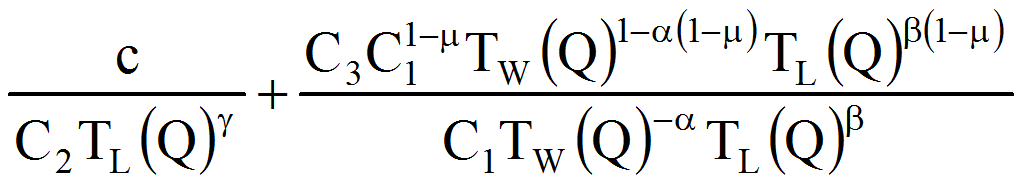=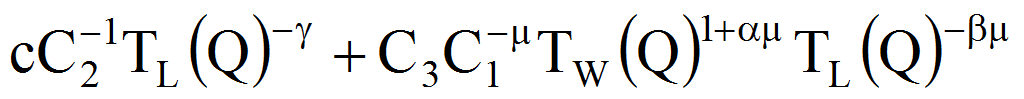=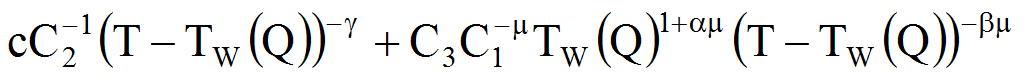therefore:

(7)=and

(8)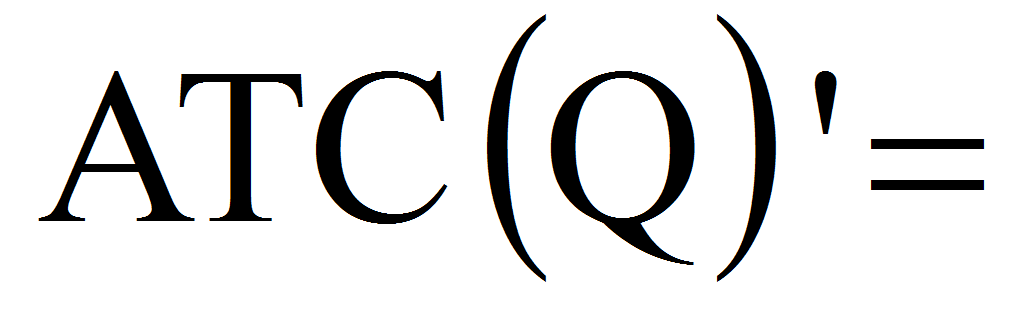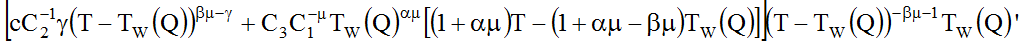From the formula of the profit: =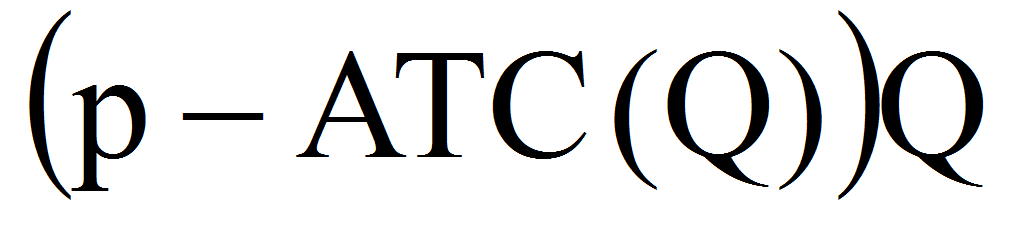follows: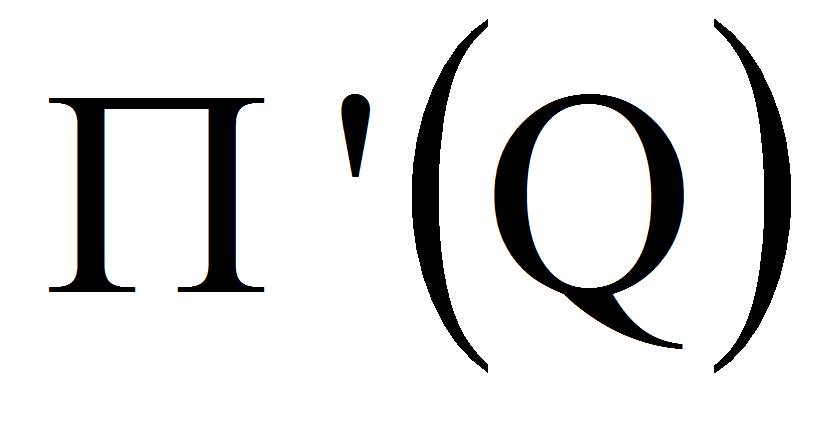=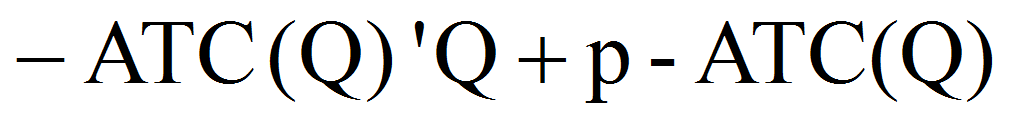The condition of profit maximization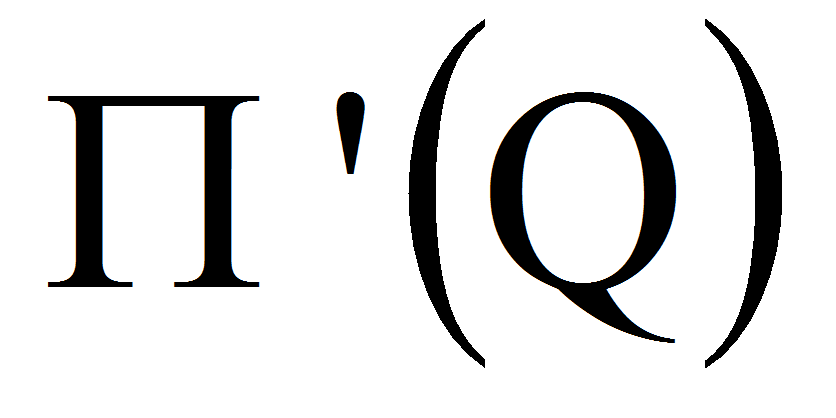=0 returns to: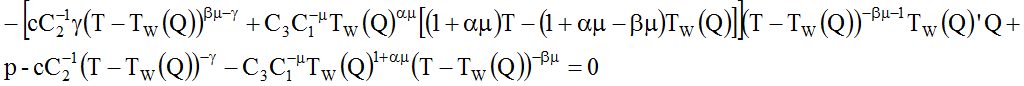from where: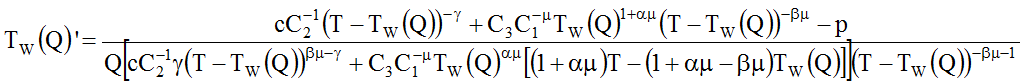Noting for simplification: 1+=, -=,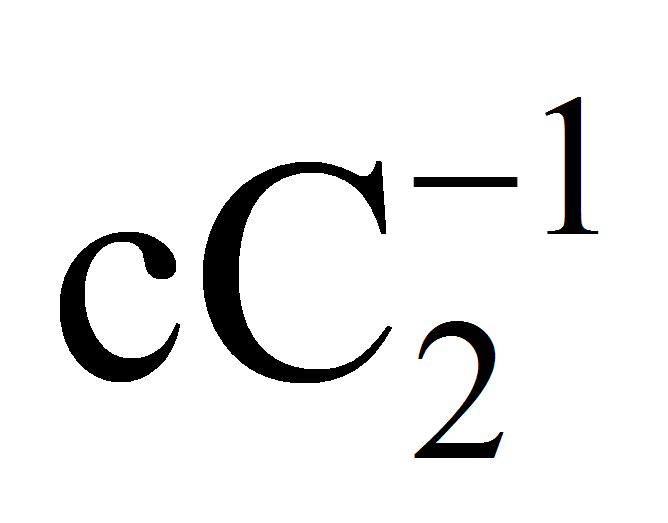=s,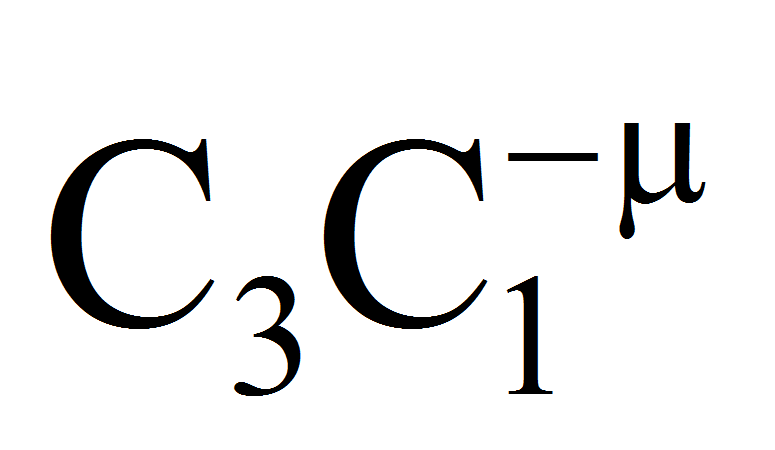=D we obtain: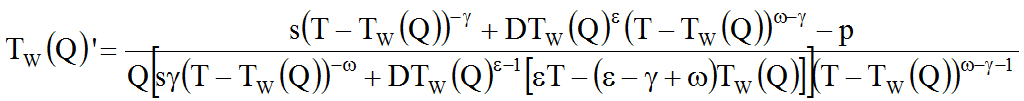or, after simplifications:

(9)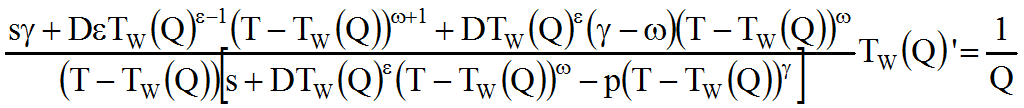Integrating with respect to Q:

(10)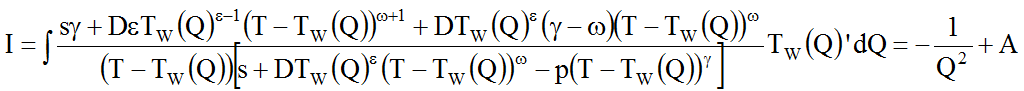,

AR.

In order to obtain the expression of TW we must compute the integral I. With the change of variable: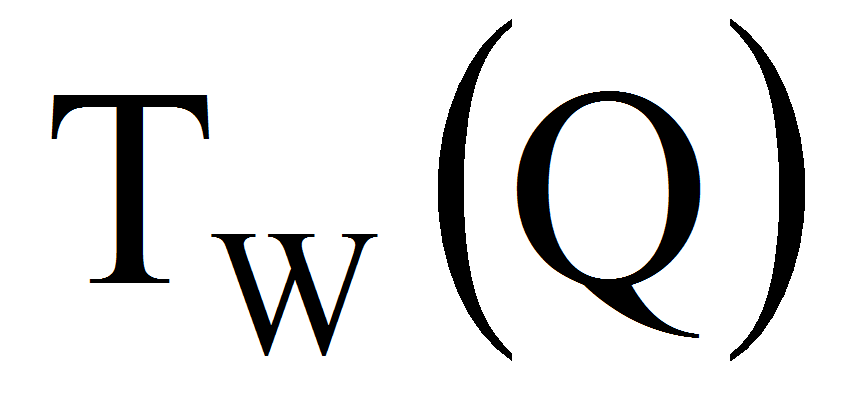=x follows: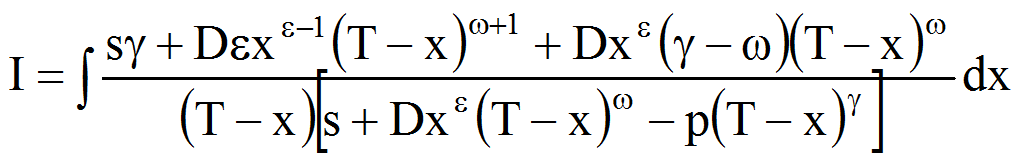=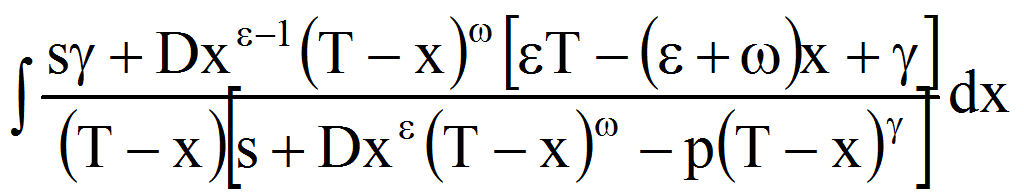If =0 that is the average productivity of capital remains constant or, in other words, it doesn’t’t inventions or innovations, we have:

(11)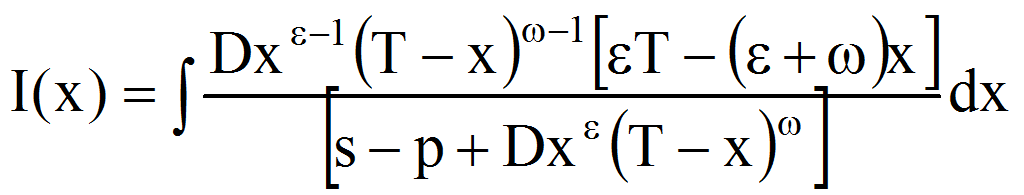With the change of variable: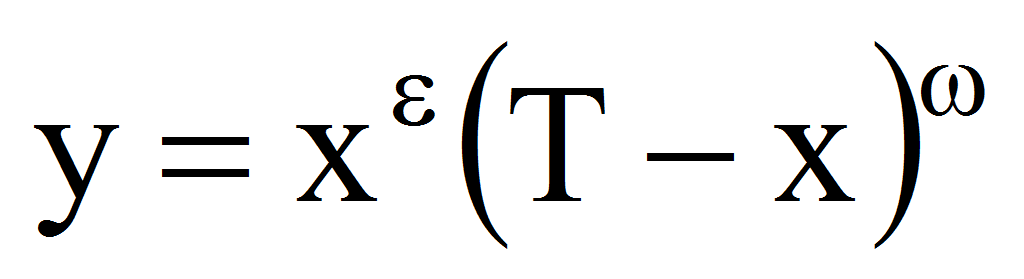in (11) we have: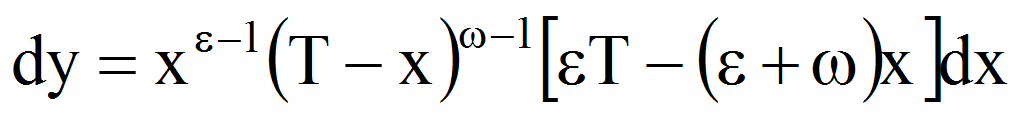therefore: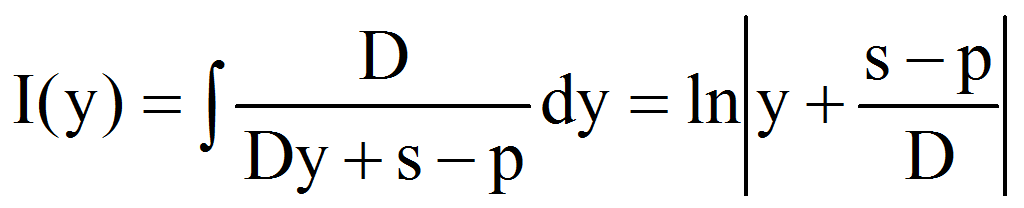or, related to the original variable:

(12)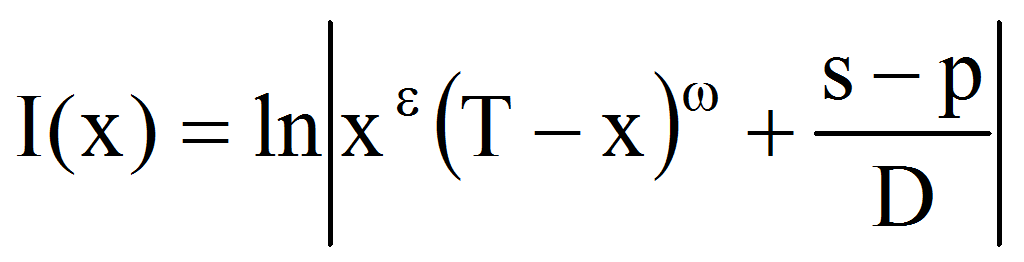The equation (10) becomes: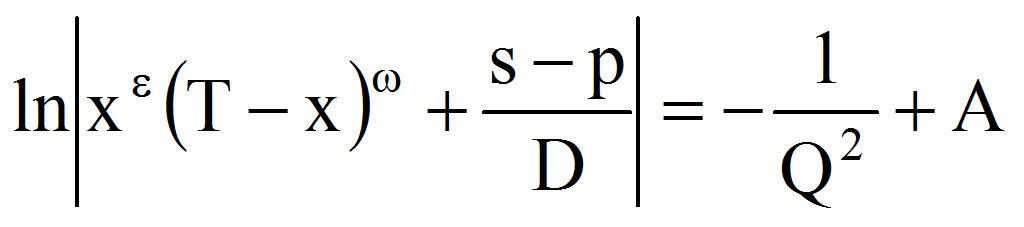or:

(13)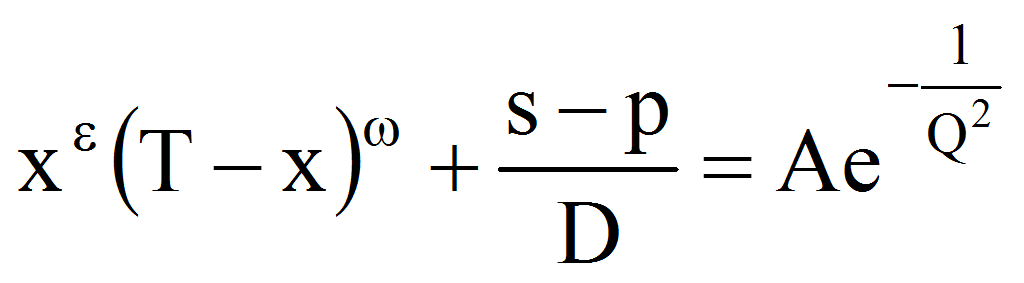, AR, x=where we note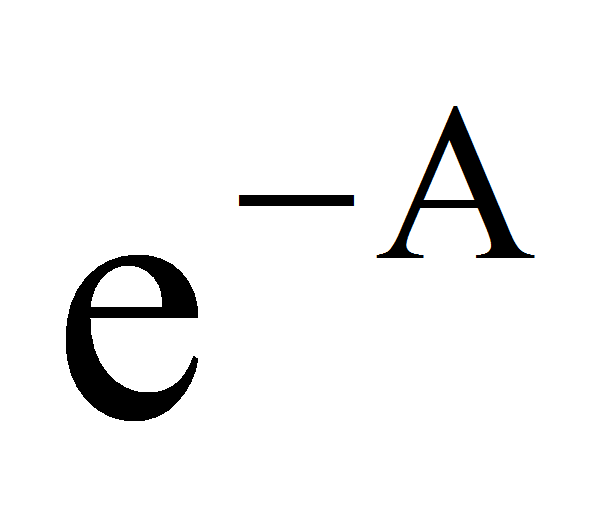with A and eliminating the modulus we have AR.

Let now the function h:(0,T)R, h(x)=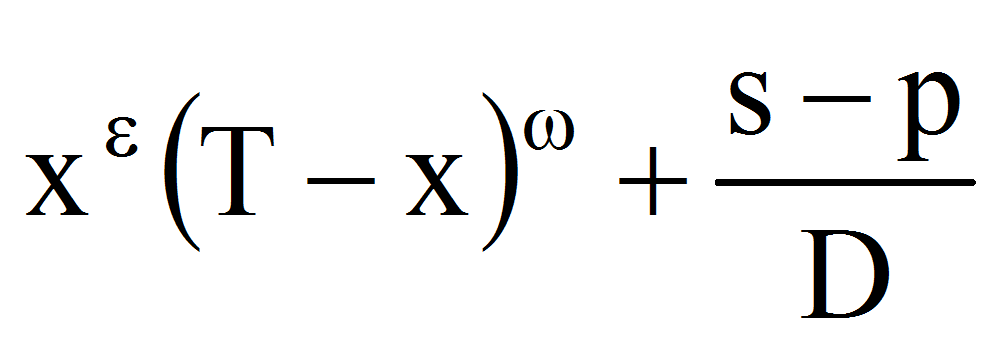.

We have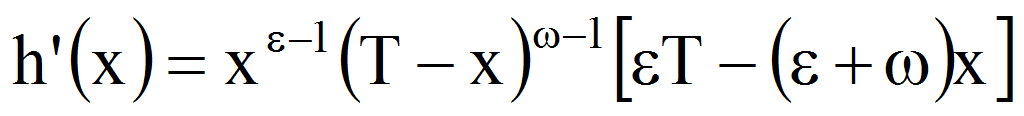. The equation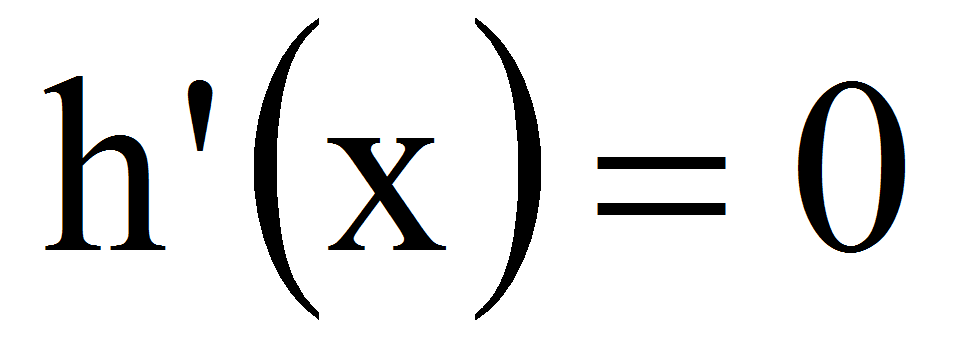implies: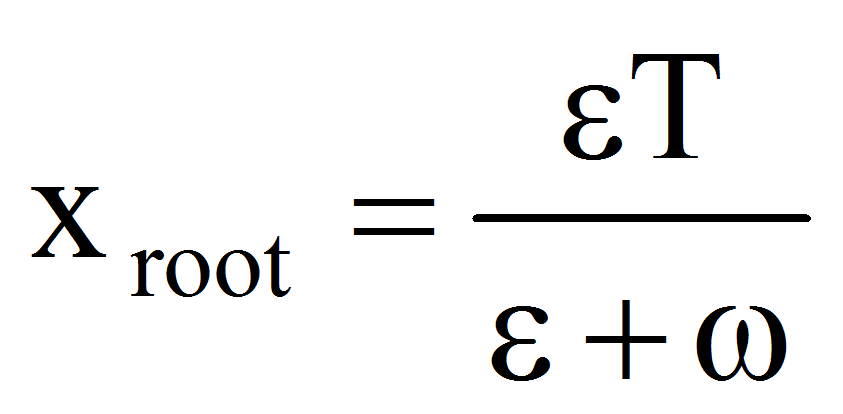How 1, 0, +=1+(-)1 follows that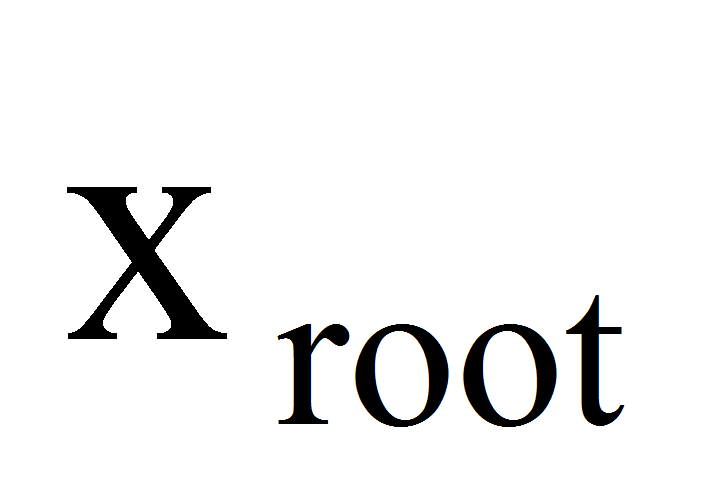(0,T) therefore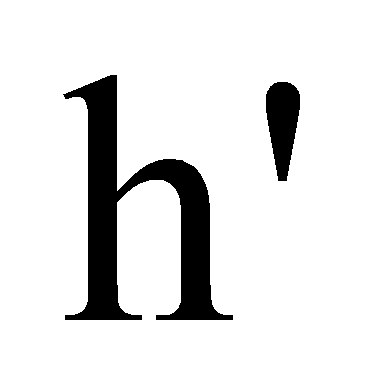has constant sign. How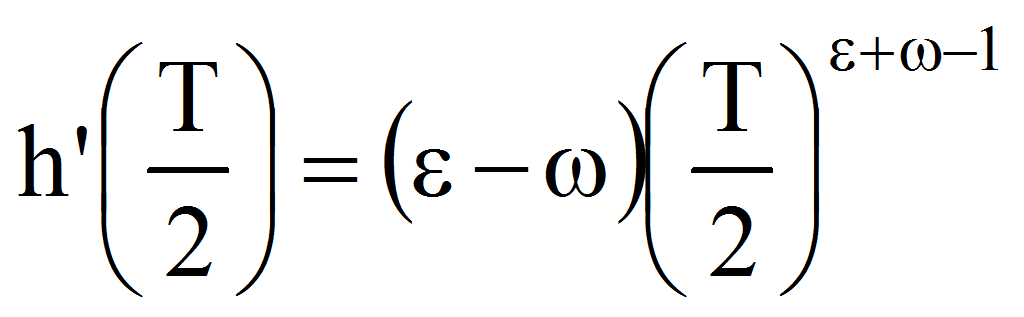0 follows that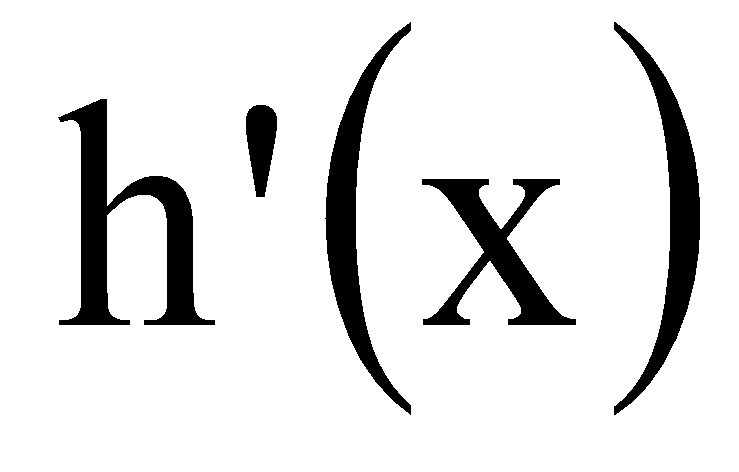0 x(0,T) therefore h is strictly increasing. Because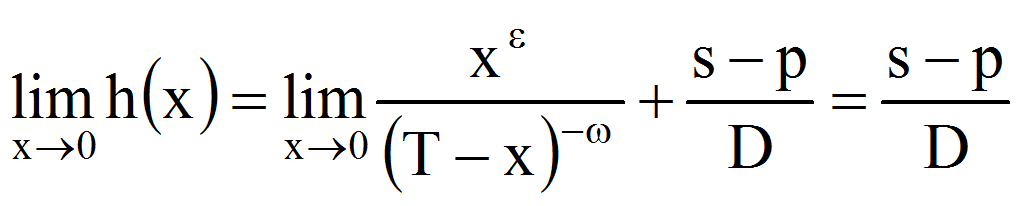and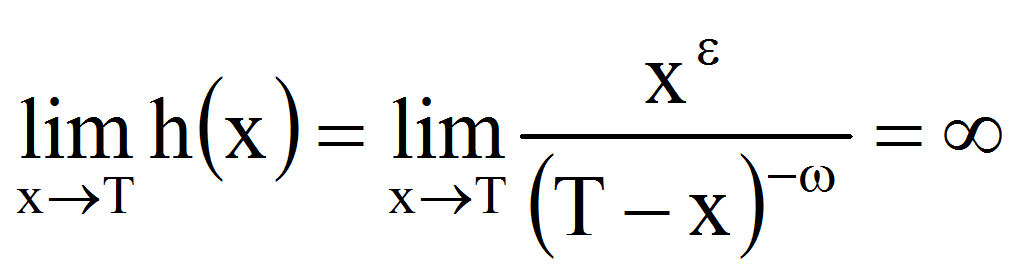follows that h:(0.T)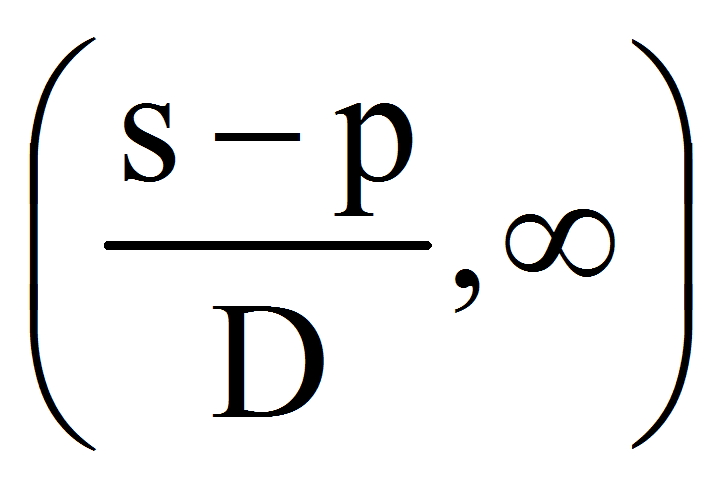is one-to-one, therefore, how the function: g:(0,)R,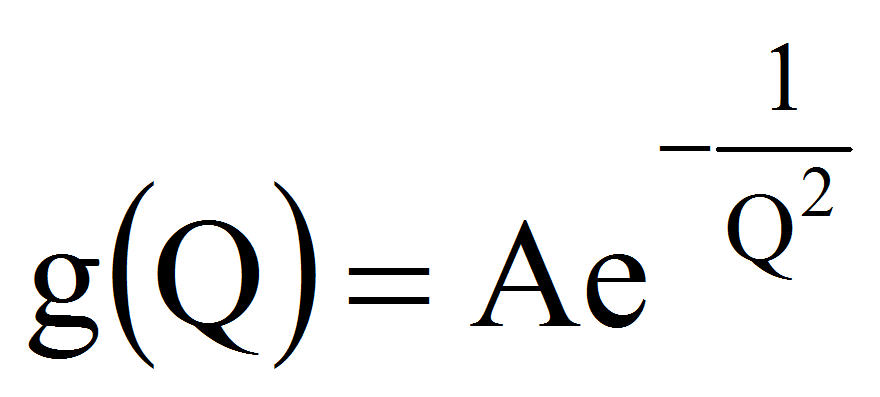has the property that Im g=(0,A) (for A0), follows:

• if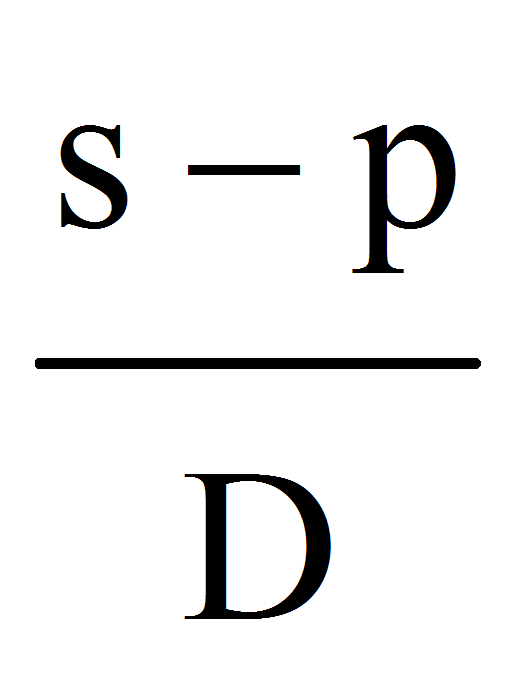0 follows that Q0 the equation:has a unique solution (for A0).

• if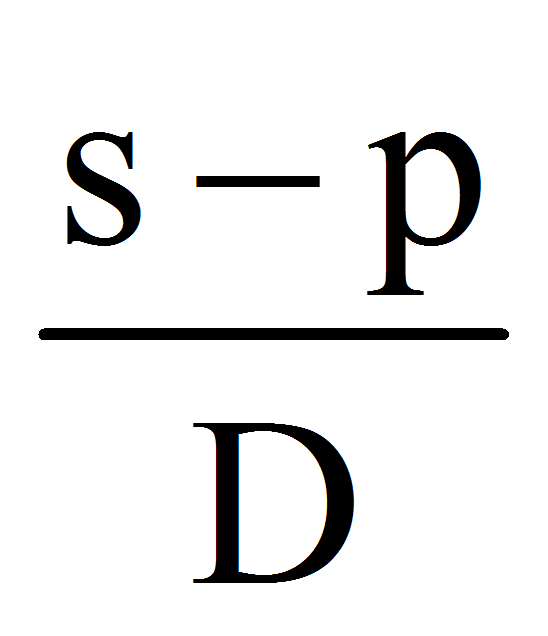(0,A) then determining Q0 from the condition (for A0):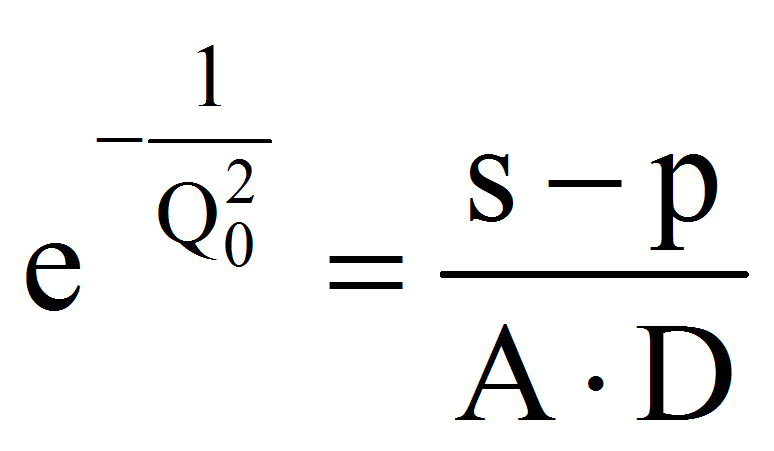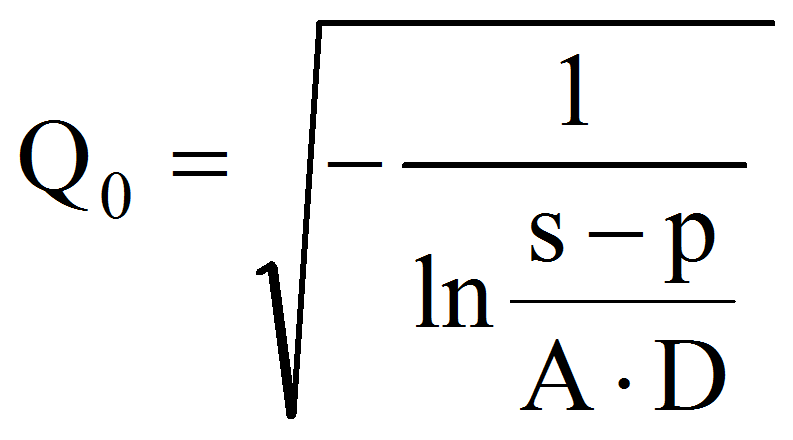follows that the equation will have an unique solution for any Q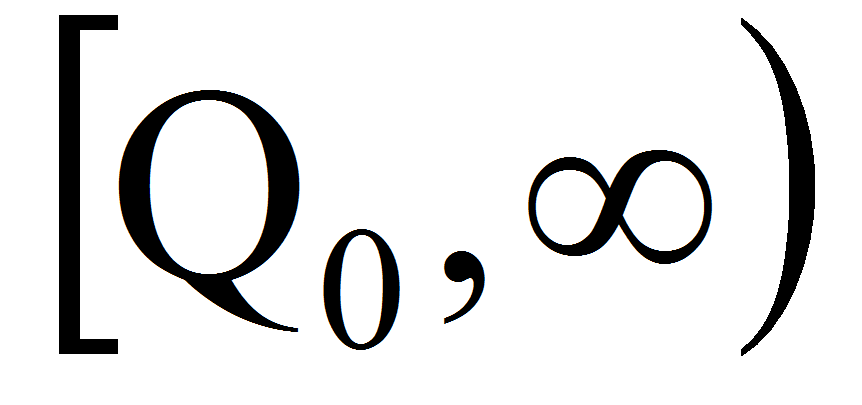.

• ifA then the equation has not solution.

Let note also that for Q0 follows that x converges to the solution of the equation: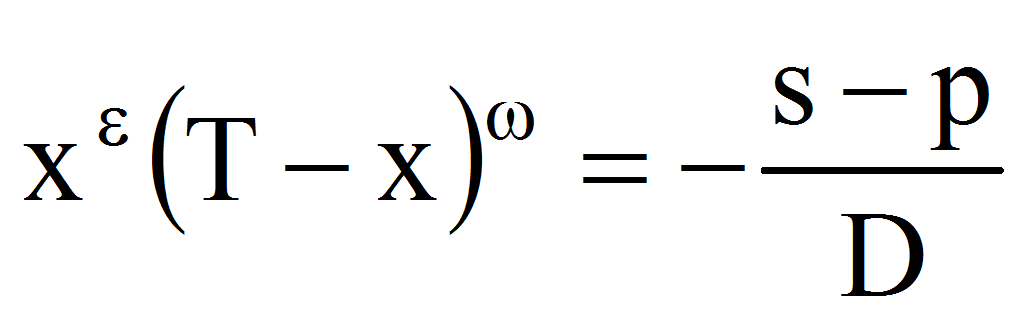. But, if for Q0 it is natural to assume that x0 results that s=p namely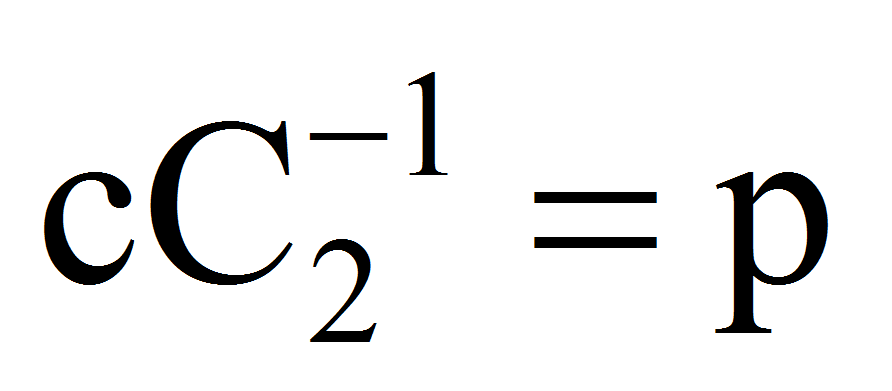therefore: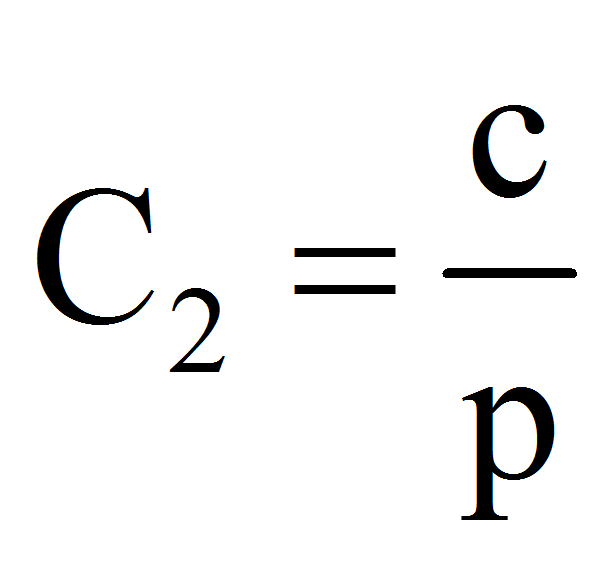.

Because the equation for the determination of x is: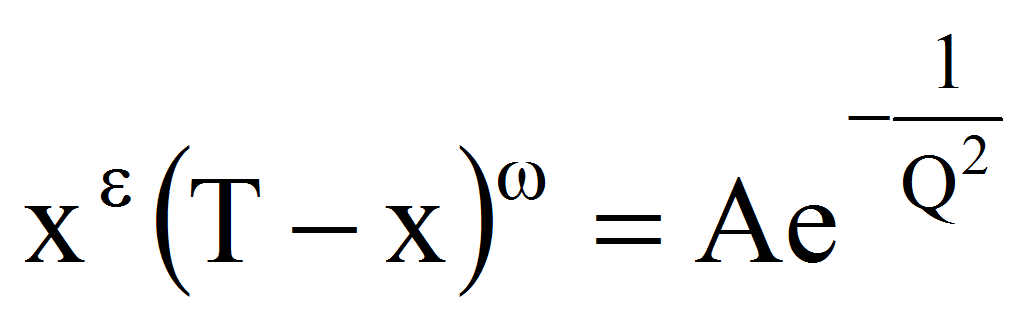we must have A0 (x(0,T)).

Because =1+, =- we finally have:

(14)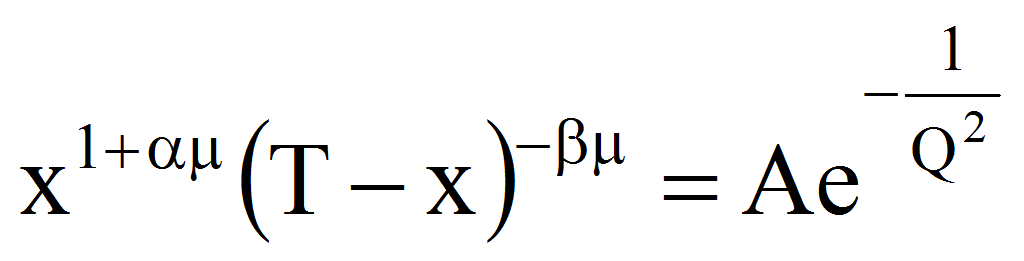, A0

defining x=TW(Q) as function of Q.

In the special case: =0 therefore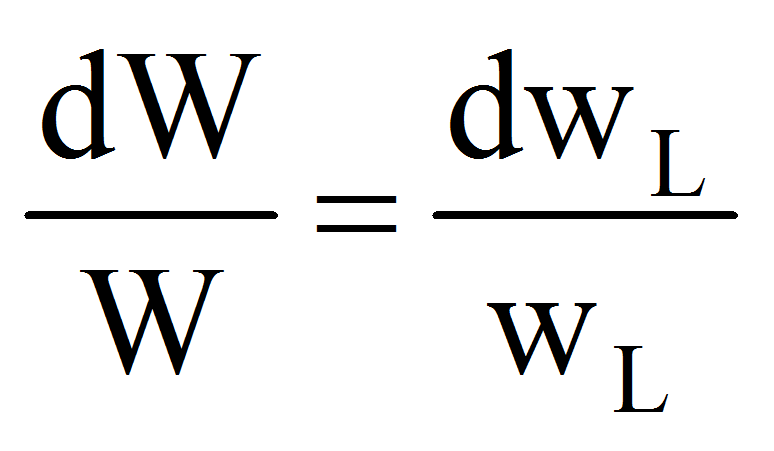that is the relative variation of wage is equal with the relative variation of the average labor productivity we obtain the equation: TW(Q)=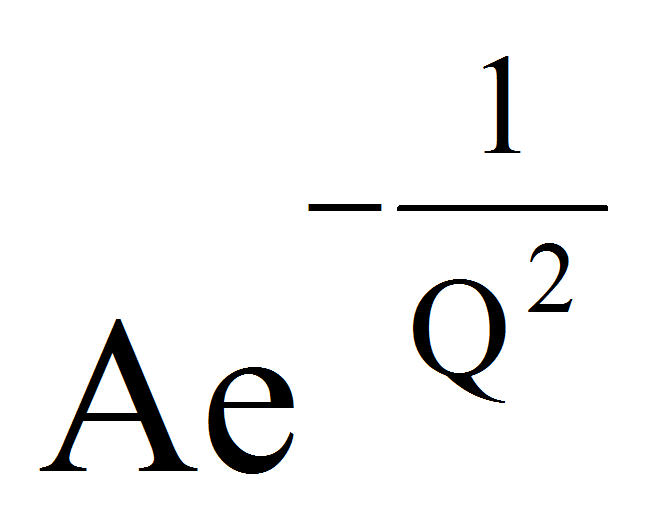.

The graph is: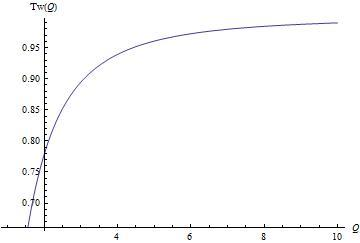Figure 1

therefore the working time increase asymptotically.

3. Conclusions

The above analysis determines the optimal balance in terms of the producer between working time and leisure. The equation of structure given by (10) determines the amount of TW for which the profit will be maximal. In fact, the computation of the integral is heavy and can be realized, in practice, after a decomposition of the function in Taylor series.

A special case when the relative variation of wage is equal with the relative variation of the average labor productivity gives us an asymptotically behavior of the working time – giving validity of the model equations structure.

4. References

Francois, P. & Roberts, J. (2003). Contracting Productivity Growth. The Review of Economic Studies, 70, pp. 59-85.

Hanf, C.H. & Müller, R. (1974). Multiple job holding and leisure time. Eur. Rev. Agric. Econ., 2, pp. 87-93.

Ladrón-de-Guevara, A.; Ortigueira, S. & Santos, M. (1999). A Two-Sector Model of Endogenous Growth with Leisure. The Review of Economic Studies, 66, pp. 609-631.

### Refbacks

• There are currently no refbacks.